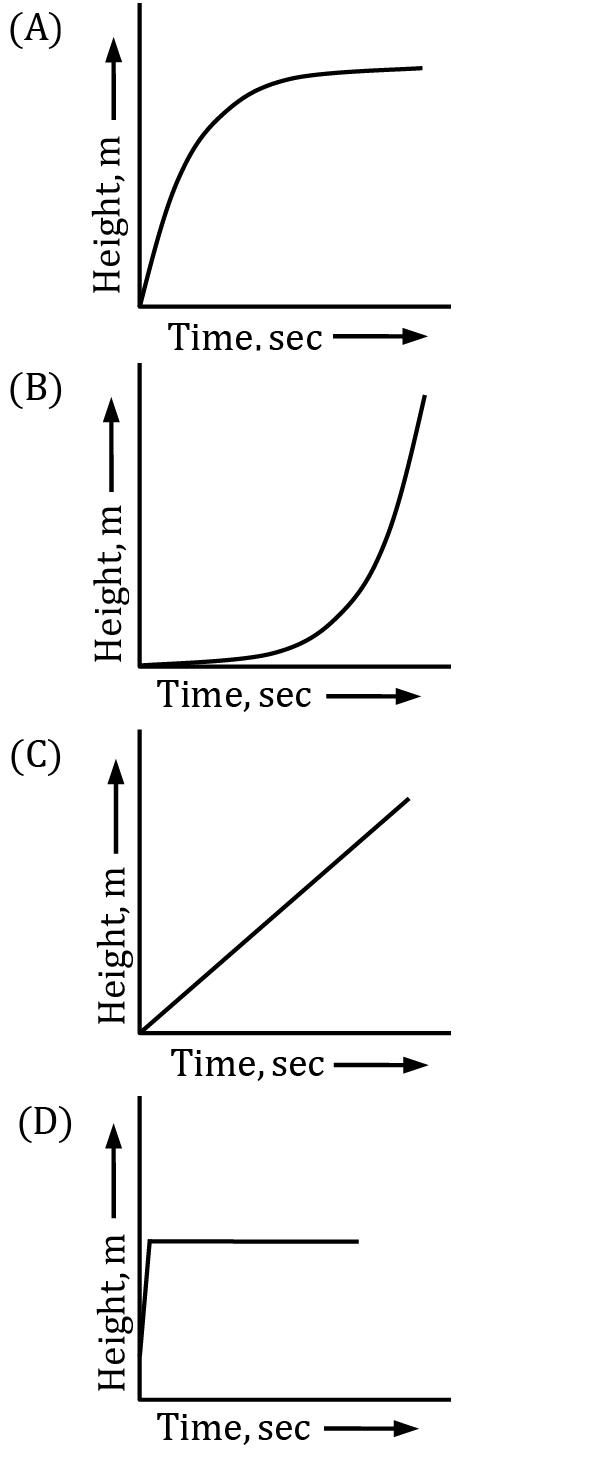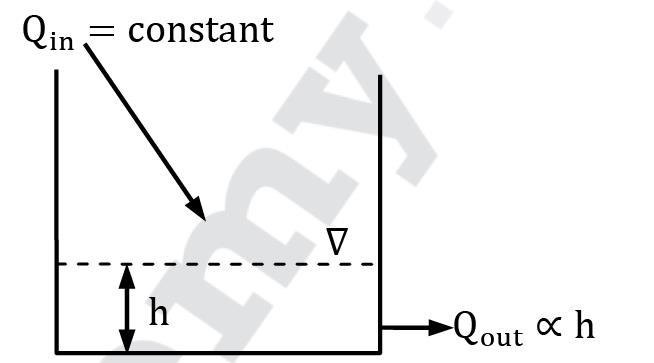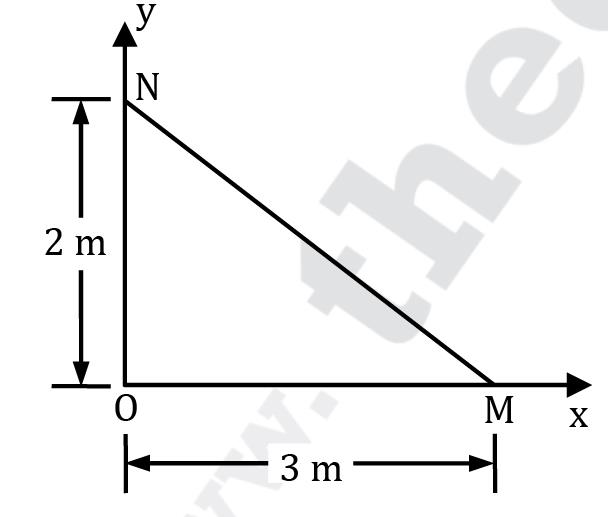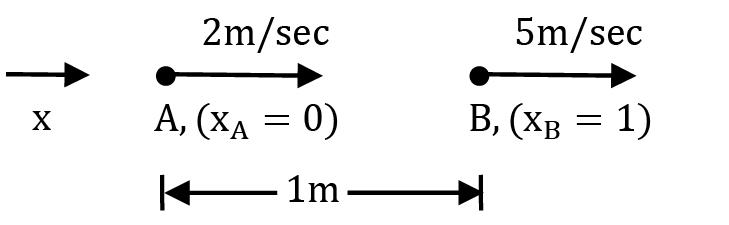Courses

# Test: Fluid Kinematics Level - 2

## 15 Questions MCQ Test Engineering Mechanics | Test: Fluid Kinematics Level - 2

Description
This mock test of Test: Fluid Kinematics Level - 2 for Civil Engineering (CE) helps you for every Civil Engineering (CE) entrance exam. This contains 15 Multiple Choice Questions for Civil Engineering (CE) Test: Fluid Kinematics Level - 2 (mcq) to study with solutions a complete question bank. The solved questions answers in this Test: Fluid Kinematics Level - 2 quiz give you a good mix of easy questions and tough questions. Civil Engineering (CE) students definitely take this Test: Fluid Kinematics Level - 2 exercise for a better result in the exam. You can find other Test: Fluid Kinematics Level - 2 extra questions, long questions & short questions for Civil Engineering (CE) on EduRev as well by searching above.
QUESTION: 1

### A 2-D flow defined by velocity field ⃗V⃗ = (x + 2y + 2)î + (4 − y)ĵ is

Solution: Given u = (x + 2y + 2) ; v = (4 − y) For the flow to be incompressible, the flow must satisfy the following continuity equation i.e ∂u /∂x + ∂v /∂y = 0

Substituting the given velocity components in LHS.

∂u /∂x + ∂v / ∂y = 1 − 1 = 0

Hence flow is incompressible.

For the flow to be irrotational, Ωz must be zero. i. e ∂v /∂x − ∂u /∂y = 0

Substituting velocity components in LHS

∂v /∂x − ∂u /∂y = 0 − (2) = −2

Hence flow is rotational or not irrotational because vorticity is non-zero.

QUESTION: 2

### A stream function is given by (x2 − y2). The velocity potential function of the flow will be

Solution: ψ = x2 − y 2

From definition of stream function (ψ)

∂ψ / ∂y = u and ∂ψ / ∂x = −v

hence u = −2y, v = −2x We also know that ∂ϕ /∂x = −u and ∂ϕ /∂y = −v

i. e ∂ϕ / ∂x = 2y and ∂ϕ / ∂y = 2x

Now dϕ = ∂ϕ / ∂x dx + ∂ϕ ∂y / dy

⟹ dϕ = 2ydx + 2xdy

ϕ = 2yx + 2xy + C

ϕ = 4(xy) + C or

ϕ = 2xy + K

where K is constant.

QUESTION: 3

### The velocity potential function of a flow field is given by x2 − y2 + constant. Its stream function will be given by

Solution: ϕ = x2 − y2 + C

∂ϕ / ∂x = −u and ∂ϕ / ∂y = −v

hence u = −2x and v = +2y

Also

∂ψ / ∂y = u ⟹ ∂ψ / ∂y = −2x ⋯ ①

and

∂ψ / ∂x = −v ⟹ ∂ψ / ∂x = −2y ⋯ ②

Partially integrating both the equations ① and ② we get

ψ = −2xy + f(x) + C1 ⋯ ③ and

ψ = −2xy + g(y) + C2 ⋯ ④

respectively.

Since both the equations ③ and ④ are same hence f(x) and g(y) will be zero and C1 = C2

∴ ψ = −2xy + constant.

QUESTION: 4

The area of a 2 m long tapered duct decreases as ! = (0.5 − 0.2x) where ‘x’ is the distance in meters. At a given instant a discharge of 0.5 m3⁄s is flowing in the duct and is found to increase at a rate of 0.2 m3 /s2. The local acceleration (in m⁄s2) at x = 0 will be

Solution: Since it is given that at a given instant, 5 m3/s of discharge is flowing in the duct, we can assume that Q = f(t) only and it is given

∂Q / ∂t = 0.2 m3/s2

Now we know that Q = A × V or V = Q / A

he local acceleration aL = ∂V / ∂t = ∂(Q⁄A) / ∂t

Since area is not dependent on time, we can write

aL = 1 / (0.5 − 0) × 0.2 = 0.4 m/s2

QUESTION: 5

Water flows into an open drum from the top at a constant mass flow rate. Water exits through a pipe near the base with a mass flow rate proportional to the height of the liquid. If the drum is initially empty, which of the following gives the plot of liquid height with time?Solution:The height (h) = Volume of fluid / Area of tank

so dh / dt = Rate of change of volume / Area of tank

Assuming Area to be constant

dh / dt = Qin − Qout / Area

dh / dt = Qin − kh / Area

Where, Qin, k and !rea are constant and at t = 0, h = 0

So clearly as h will increase, dh / dt will decrease i.e as h increases, slope of h-T curve will decrease.

QUESTION: 6

A stream function is given by ψ = 4x2y + (2 + t) y2 Find the flow rates across the faces of the triangular prism OMN (Fig.) having a thickness of 5 m in the z-direction at the time instant t = 2 seconds. (in m3/s)Solution: ψ = 4x2y + (2 + t)y2

The coordinates of point N are (0, 2)

∴ ψN = 0 + (2 + 2) × 22 = 16 The co-ordinates of point M are (3, 0)

∴ ψM = 0 + 0 = 0 The coordinates of point O are (0, 0)

∴ ψO = 0 + 0 = 0

Flow rate across face NO

= 5 (ψN − ψO)

= 5 (16 − 0) = 80 m3⁄s

Flow rate across face MO

= 5 (ψM − ψO)

= 5(0 − 0) = 0 m3⁄s

Flow rate across face NM

= 5(ψN − ψM)

= 5 (16 − 0)

= 80 m3⁄s

QUESTION: 7

For a two-dimensional potential flow, the velocity potential function is given by ϕ = 4x(3y − 4). The numerical value of stream function at the point (2, 3) is

Solution: Given ϕ = 12xy − 16x ∂ϕ /∂x = −u and ∂ϕ /∂y = −v

∴ u = 16 − 12y & v = −12x

Also dψ = ∂ψ / ∂x dx + ∂ψ /∂y dy

and ∂ψ ∂x = −v and ∂ψ ∂y = u

∴ dψ = 12xdx + (16 − 12y)dy

ψ = 12x2 2 + 16y − 12y2 2

ψ = 6x2 + 16y − 6y2

Hence at point (2, 3) ψ = 6(2)2 + 16(3) − 6(3)2 ψ = 18 units

QUESTION: 8

In a stream line steady state flow, two points A and B on a stream line are 1 m apart and the velocity of a particle varies linearly from 2 m/s to 5 m/s as the particle moves from A to B. What is the acceleration of fluid at B?

Solution:For a flow a = U ∂U / ∂x +∂U /∂t

∂U / ∂t = 0

Also it is given, that as particle moves from A to B its velocity increases linearly,

therefore U = ax + b And

hence

∂U/∂x =UB − UA / xB − xA = 5 − 2 / 1 − 0 = 3/sec

Thus, a(x) = U(x) × 3 m/sec Hence a at B

a(xB) = U(xB) ∂U /∂x

a(xB) = 5 × 3

a(xB) = 15 m/sec

QUESTION: 9

Statement I: When flow is unsteady, both normal and tangential components of acceleration will occur.

Statement II: During unsteady flow, in addition to the change of velocity along the path, the velocity will also change with time.

Solution: The normal acceleration in the flow represents the motion of fluid particles along curved paths (e.g. flow through bends) and tangential acceleration represents the increase in speed of the particle as it moves along the path. These two acceleration components can exist even if the flow is steady. Example: low of water through a converging bend at constant rate.

Also consider flow of water at an increasing or decreasing flow rate through a straight pipe of uniform cross-section. In this case normal acceleration is zero, even though tangential acceleration is non-zero and a function of time, making the flow unsteady.

QUESTION: 10

Statement I: Non-viscous flow between two plates held parallel with a very small spacing between them is an example of irrotational flow.

Statement II: Forced vortex implies irrotational flow.

Solution: For non-viscous flow between two-parallel plates, v and w will not exist as flow is in one direction, so only u will exist. As no viscous effects are present ∂u⁄∂y = 0. Also v = 0 hence ∂v⁄∂x = 0. Thus the flow is irrotational.

A forced vortex represents a rotational flow. Hence statement (II) is false.

QUESTION: 11

The stream function for a flow field is ψ = 3x2 y + (2 + t)y2. The velocity at a point P for position vector r = î + 2ĵ − 3k̂ at time t = 2 will be

Solution: ψ = 3x2y + (2 + t)y2

By definition of ψ ; ∂ψ /∂y = u and ∂ψ/ ∂x = −v

Therefore u = 3x2 + 2(2 + t)y and v = −6yx

Thus the velocity vector is

⃗V = uî + vĵ

⃗V = [3x2 + 2(2 + t)y]î − 6xyĵ

at r = î + 2ĵ − 3k̂ , i. e. (1, 2, −3)

and t = 2

V = [3(1)2 + 4(2 + 2)]î − 6(1 × 2)ĵ

V = 19î − 12ĵ

QUESTION: 12

In a two-dimensional incompressible steady flow, the velocity component u = !ex is obtained. What is the other component v of velocity?

Solution: For a 2D incompressible flow

∂u/ ∂x + ∂v / ∂y = 0 ⋯ ①

Given u = Aex Therefore ∂u / ∂x = Aex

Substituting the same in equation ①

Aex + ∂v / ∂y = 0

⇒∂v / ∂y = −Aex

⇒ v = −Aex y + g(x)

QUESTION: 13

The instantaneous velocity and density fields for flow through a diffuser are given as u = u0 e−2x/L and ρ = ρ0 e−x/L Find the rate of change of density for the same time instant at x = L.

Solution: Considering continuity equation for linear flow

∂ρ / ∂t + ∂(ρu) / ∂x = 0

∴ ∂ρ / ∂t = −∂(ρu) / ∂x

Consider the RHS of the above equation.

As it is a partial derivative with respect to space co-ordinate, time terms will be treated as constants. Hence substituting instantaneous density field and velocity field in RHS we get instantaneous rate of change of density.

∂ρ /∂t = −∂ / ∂x (ρ0e−x/L u0e−2x / L)

∂ρ / ∂t = −∂ / ∂x (ρ0 u0e −3x /L )

Therefore at x = L

∂ρ / ∂t = 3 / L ρ0 u0 e-3

QUESTION: 14

State whether the following flow field is physically possible? u = 3xy2 + 2x + y2 and v = x2 − 2y − y3

Solution: Checking for incompressible continuity equation i.e

⇒∂u / ∂x + ∂v / ∂y = 3y2 + 2 − 2 − 3y2 = 0 The flow satisfies incompressible continuity equation, so it is incompressible.

Since u and v are not functions of time, flow is steady. Also

∂v / ∂x − ∂u / ∂y = 2x − 6xy − 2y

i.e vorticity is non-zero.

Thus, flow is rotational.

QUESTION: 15

Which of the following statements is correct in respect of velocity potential function & stream function?

1. Stream function is defined only for an incompressible irrotational flow

2. Velocity Potential function is defined only for an incompressible irrotational flow

3. If the stream function exists it shall definitely satisfies the Laplace equation

4. If the velocity potential function exists it shall definitely satisfies the Laplace equation

Solution: A stream function is defined only for incompressible flow whether it is rotational or irrotational. However it satisfies the Laplace equation only when flow is irrotational.

A velocity potential function is defined only for irrotational flow whether it is compressible or incompressible. However it satisfies the Laplace equation only when flow is incompressible.